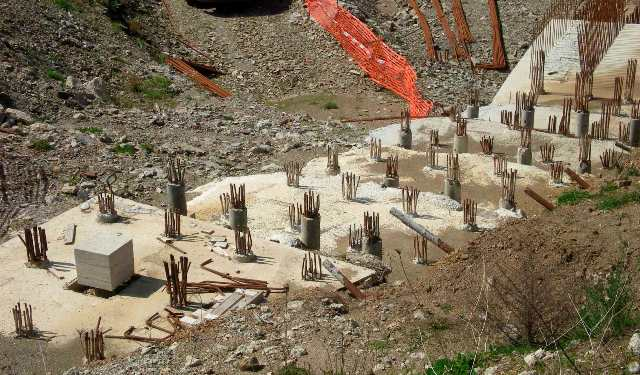# HOW TO CALCULATE PILE LOAD CAPACITY USING STATIC CONE PENETRATION TEST?## Pile Load Capacity Calculation Using Static Cone Penetration (SCPT) Test Data

The safe load capacity of bored cast in situ piles can be calculated using the test data obtained from static cone penetration test as described below.

We know that the ultimate load capacity of pile consist of two terms, i.e.

• End bearing resistance
• Skin friction resistance

So we need to calculate the above two parameters, thereafter by adding these two parameters we can obtain the ultimate load capacity of pile.

### Step-1 (Calculate End-bearing Resistance of Pile)

The ultimate end bearing resistance of pile is calculated using the formula given below.Where,

qu = Ultimate end bearing resistance, in kN/m2

qc0 = Average static cone resistance over a depth of 2D below the pile tip, in kN/m2

qc1 = Minimum static cone resistance over the same 2D below the pile tip, in kN/m2

qc2 = Average of the envelope of minimum static cone resistance values over the length of pile of 8D above the pile tip, in kN/m2

D = Diameter of pile

### Step-2 (Calculate Skin-friction Resistance)

For different types of soils, the ultimate skin friction resistance (fs), in kN/m2, can be obtained using the table given below.

 Type of soil Skin friction resistance (fs), in kN/m2 qc less than 1000 kN/m2 qc/30 to qc/10 Clay qc/25 to 2qc/25 Silty sand & silty clay qc/100 to qc/25 Sand qc/100 to qc/50 Coarse sand & gravel qc/150 to qc/100

Where, qc is cone resistance in kN/m2.

### Step-3 (Calculate Safe Pile Load Capacity)

Add the ultimate end bearing resistance (qu) and ultimate skin friction resistance (fs) as calculated in step-1&2 respectively, and divide it with a suitable factor of safety (minimum 2.5) to get the safe load capacity of pile, i.e.

Qsafe = (qu+fs) / F.O.S.

Where,

Qsafe = Safe load capacity of pile

qu = Ultimate end bearing resistance of pile

fs = Ultimate skin friction resistance of pile

F.O.S. = Factor of safety of pile (usually 2.5)

### Reference

IS: 2911-Part-1-Sec-2

One Comment
1.